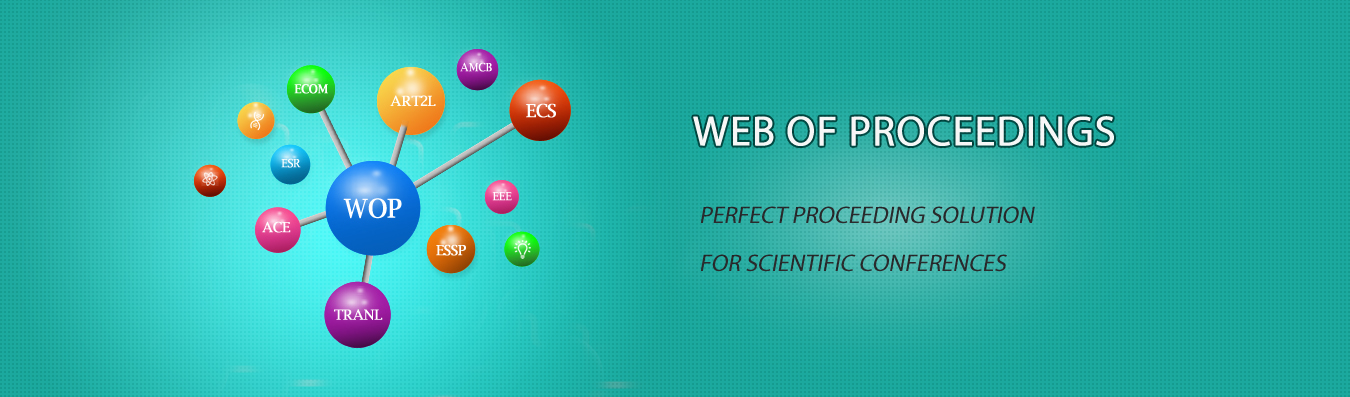The best way to conference proceedings by Francis Academic Press### Study of Reasoning Rules in Discrete Mathematical Propositional Calculus

DOI: 10.25236/icme.2019.129

Shao Jingjing

Shao Jingjing

#### Abstract

Mathematical logic is the science of reasoning, especially in mathematics. In the process of logical reasoning, if there are more propositional arguments, it is not convenient to use the column truth table or equivalent calculus, so the method of constructing proof should be introduced. This proof method must be carried out under the given rules, which requires students to have a high degree of abstract thinking ability and rigorous mathematical reasoning skills. The important rules of reasoning are the core of this part. Only by exploring the rules can we master the reasoning system well. Mathematical logic has two main parts: propositional calculus and predicate calculus. The axiom system of the perpetual truth formula in propositional calculus is a system that gives a number of perpetual truth formulas (or rules of reasoning) and then gives a number of rules of reasoning derived from the perpetual truth formula from which all the perpetual truth formulas are derived. The reasoning theory of proposition should master important reasoning rules, make clear the formal structure of reasoning, master the method to judge whether reasoning is correct and apply rules, rules and rules . The difficulty of mathematical logic is the reasoning theory and application, which should first find the learning method of the important reasoning rules in propositional calculus.

#### Keywords

Propositional calculus; Rules of reasoning; Hypothetical reasoning; Modus tollendo ponens